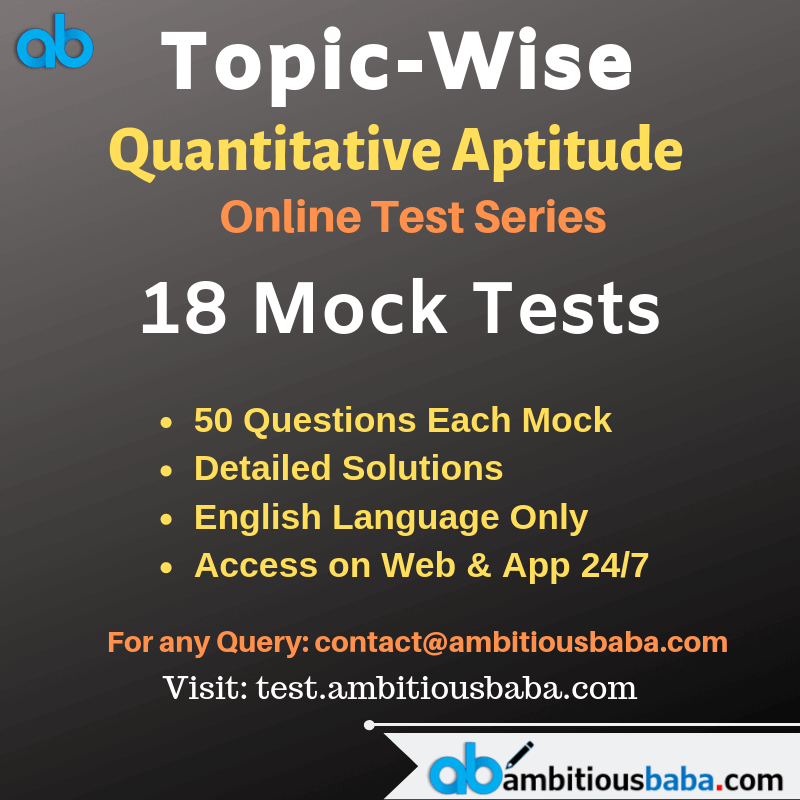# Quant Quiz for SBI CLERK Mains & RBI Assistant Mains 2020 |7 May 2020

## Quant Quiz SBI CLERK MAINS & RBI ASSISTANT MAINS

Quant Quiz to improve your Quantitative Aptitude for SBI Po & SBI clerk exam IBPS PO Reasoning , IBPS Clerk Reasoning , IBPS RRB Reasoning, LIC AAO ,LIC Assistant  and other competitive exams

Q1. Veer can complete a work in X days and Ayush is 40% more efficient than Veer. If both completeof work together and get Rs. 1200 for it, then find difference between wage share of Veer and Ayush?
1. 240 Rs.
2. 180 Rs.
3. 160 Rs.
4. 200 Rs.
5. 400 Rs.
Q2. A boat covers 11.2 km in downstream in 24 minutes and ratio of speed of boat in still water to speed of stream is 5 : 2. Find ratio of time taken by boat to cover 27 km in upstream to time taken by boat to cover 105 km in downstream?
1. 5 : 3
2. 3 : 5
3. 3 : 4
4. 2 : 3
5. 1 : 3
Q3. If sum of length of two rectangles A & B is 30 cm and their breadths are 8 cm and 6 cm respectively. If ratio of perimeter of A to B is 7 : 4, then find difference between perimeters of these two rectangles?
1. 24 cm
2. 16 cm
3. 36 cm
4. 18 cm
5. 12 cm
Q3. Anurag invested Rs. 800 at the rate of ‘R’ % at SI for three years and Ayush invested Rs. 1200 at the rate of (R – 2.5) % at SI for three years. If interest received by Ayush is Rs. 60 more than Anurag, then find ‘R’%?
1. 15%
2. 12.5%
3. 10%
4. 8%
5. 7.5%
Q5. Anurag a general store owner wants to make some profit by selling wheat. He used various methods, which of the following would maximize his profit ? I. Sell wheat at 15% profit. II. He cheats and uses 150 gm less weight instead of 1 kg and sold it at cost price?
1. I
2. II
3. None of these
4. Profits are same for I and II
5. can’t be determined

Q6. A person invested a certain amount at simple interest at the rate of 6 per cent per annul earning Rs. 900 as an interest at the end of three years. Had the interest been compounded every year, how much more interest would he have earned on the same amount with the same interest rate after three years ?

(a) Rs. 38.13

(b) Rs. 25.33

(c) Rs. 55.08

(d) Rs. 35.30

(e) None of these

Q7.  If Rs.1000 be invested at interest rate of 5% and the interest be added to the principle every 10 years, then In how many years  it will amount to Rs. 2000 ?Q8. If diameter of the circle is equal to the side of the square having perimeter 112 cm then find the difference between area of the square and that of the circle?
1. 180 cm
2. 178 cm²
3. 168 cm²
4. 154 cm²
5. 152 cm²
Q9. Population of a village in 2015 was 36,000. There was a successive increment of 17.5% and 20% in 2016 and 2017 respectively. Find the population at the end of 2018 if there was a decrement of 25% in 2018?
1. 39150
2. 36270
3. 37140
4. 39300
5. 38070
Q10. A bat was sold at Rs 1600. If it were sold at 200 more than the profit percent will be 16% more then find the cost price of the bat?
1. Rs 1250
2. Rs 1200
3. Rs 1000
4. Rs 900
5. Rs 1050

## Solutions

Q1. Ans(4)Q2. Ans(2)
Let speed of boat in still water and speed of stream be 5x km/hr and 2x km/hr respectivelyQ3. Ans(1)Q4. Ans(2)Q5. Ans(2)6. Ans(c)S7. Ans(a)Q8 Ans(3)Q9. Ans(5)Q10. Ans(1)### Online Mock Test Available on App as well as Web:### How to Access on App:-

1. Go to Playstore search Ambitious Baba or Click here to Install App
2. After Install Login with Google Account or Facebook Account

### You Can Read This Also:

ambitiousbaba.com need your support to Grow

I challenge you will get Best Content in Our PDFs with Detail solutions and Latest Pattern

Memory Based Puzzle E-book | 2016-19 Exams Covered

Get PDF here

Caselet Data Interpretation 200 Questions

Get PDF here
Puzzle & Seating Arrangement E-Book for BANK PO MAINS (Vol-1)

Get PDF here

### ARITHMETIC DATA INTERPRETATION 2019 E-book

Get PDF here
The Banking Awareness 500 MCQs E-book| Bilingual (Hindi + English)

Get PDF here

High Level DATA INTERPRETATION Practice E-BOOK

Get PDF her

3